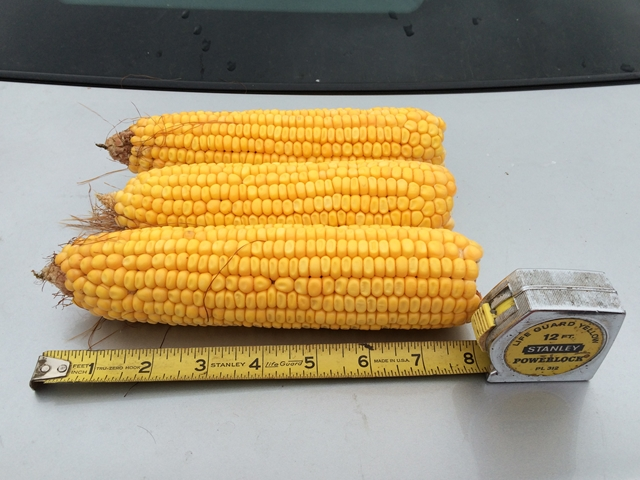# 7 Steps for Estimating Corn Yields( AgWeb )

A 55-mph drive by your cornfields won’t cut it if you want to avoid surprises at the end of the season. Lace up your scouting boots and get into each of your fields to estimate yield.

Each year, Farm Journal sends a team into corn and soybean fields to estimate yields before the end of the season. Keep up with their findings here. Follow these seven steps to calculate corn yield like the pros on this year’s Farm Journal Midwest Crop Tour.

1. Measure and record the row spacing (inches) used in the field.
2. Walk through the end rows into the bulk of the field, then walk 35 paces down the rows to the first sampling area.
3. Measure 30' down the row, then count all ears in the two adjacent rows. Divide that number by two and record it. For example: (42 ears in one row 45 ears in other row) ÷ 2 = 43.5 ears
4. Pull the fifth, eighth and 11th ears from plants in one row of the sampling area.
5. Measure length of the portion of each ear that successfully developed kernels. Calculate the average ear length of the three ears and record it. For the most accurate estimate, sample fields in late kernel dough stage or even kernel dent stage. For example: (6" 7" 5") ÷ 3 = 6"
6. Count the number of kernel rows on each ear. Calculate the average kernel row number and record it. For example: (16 rows 14 rows 16 rows) ÷ 3 = 15.3 rows
7. Grain yield for the sampling area is calculated by multiplying the average ear count by the average ear length by the average kernel row number, then dividing by the row spacing. For example: (43.5 ears x 6" x 15.3 rows) ÷ 30" rows = 133 bu. per acre yield estimate
Tags: